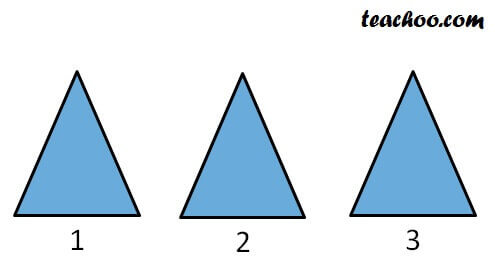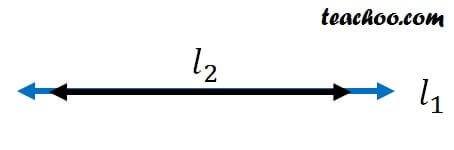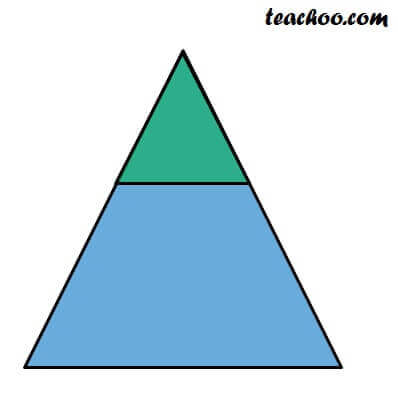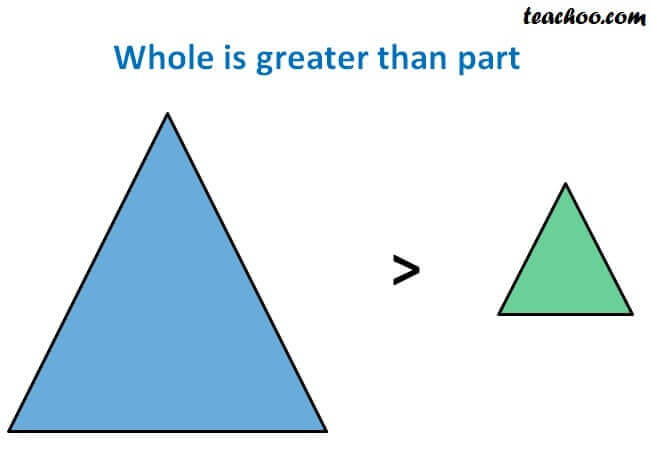Axioms

Chapter 5 Class 9 Introduction to Euclid's Geometry
Concept wise

Some of Euclid’s axioms are:

1. Things which are equal to the same thing are equal to one another.
2. If equals are added to equals, the wholes are equal.
3. If equals are subtracted from equals, the remainders are equal.
4. Things which coincide with one another are equal to one another.
5. The whole is greater than a part.
6. Things which are double of the same things are equal to one another.
7. Things which are halves of the same things are equal to one another.

## 1 st Axiom

1st axiom says Things which are equal to the same thing are equal to one another.

An application of 1 st axiom can be

Area of triangle 1 = Area of triangle 2

& Area of triangle 3 = Area of triangle 2

So, Area of triangle 1 = Area of triangle 3## 4 th axiom

4 th axiom says two things as identical. Then they must be equal.

Since line l 1 and l 2 are identical.

They are equal## 5 th Axiom

5 th axiom is The whole is greater than a part.

This axiom gives us the definition of greater thanHere,

Area of Blue triangle is greater than the area of green triangle.Learn in your speed, with individual attention - Teachoo Maths 1-on-1 Class